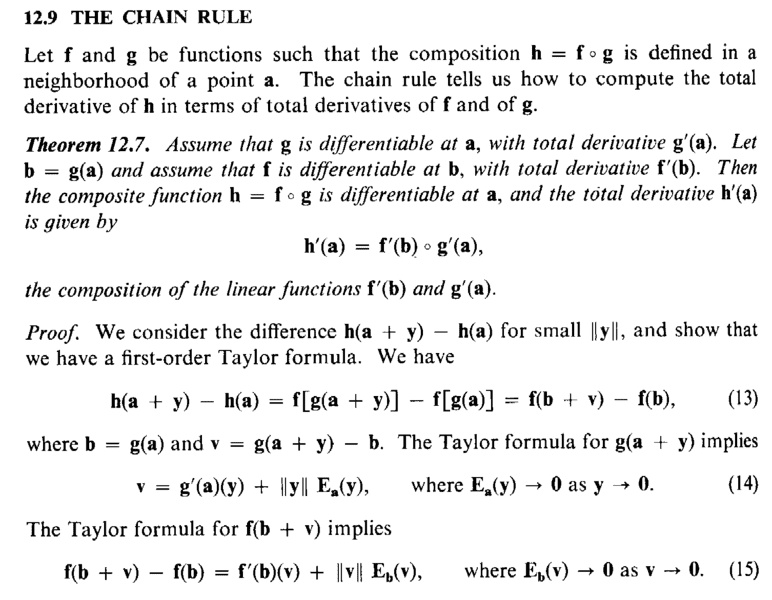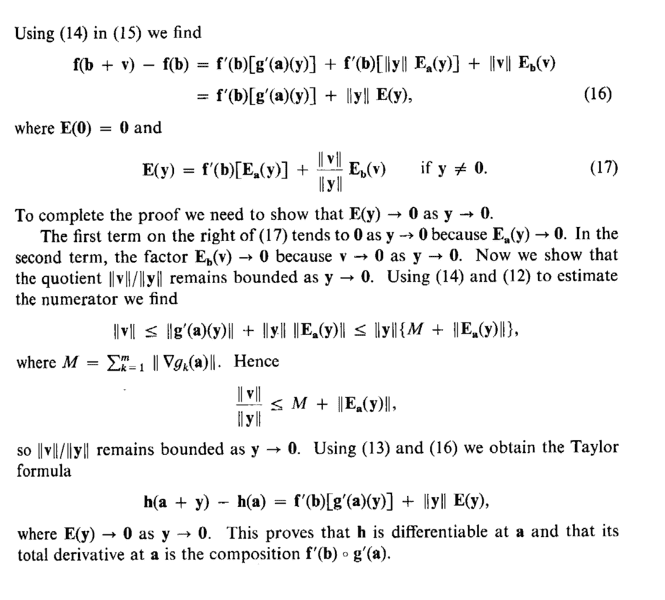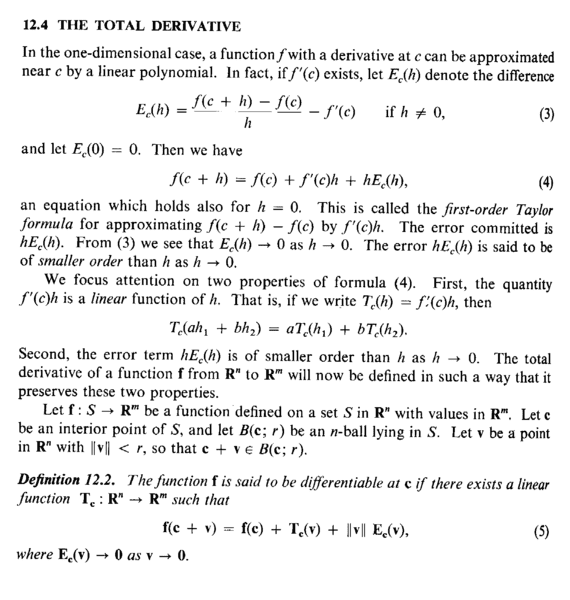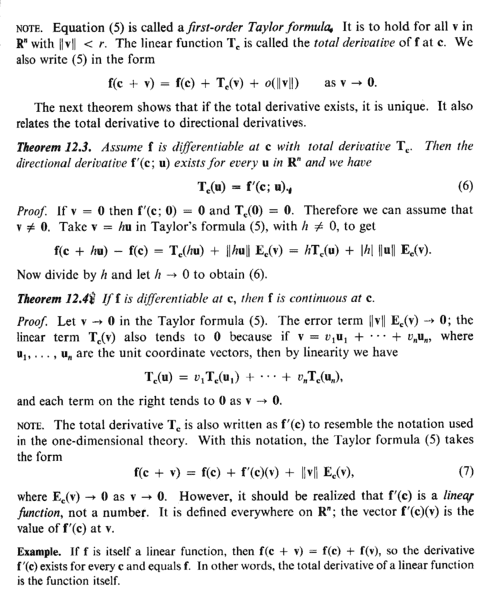# The Chain Rule for Multivariable Vector-Valued Functions ...

Gold Member
I am reading Tom M Apostol's book "Mathematical Analysis" (Second Edition) ...

I am focused on Chapter 12: Multivariable Differential Calculus ... and in particular on Section 12.9: The Chain Rule ... ...

I need help in order to fully understand Theorem 12.7, Section 12.9 ...

Theorem 12.7 (including its proof) reads as follows:In the proof of Theorem 12.7 we read the following:

" ... ... Using (14) in (15) we find

##f(b+v) - f(b) = f'(b) [ g'(a) (y) ] + f'(b) [ \| y \| E_a(y) ] + \|v \| E_b(v)##

##= f'(b) [ g'(a) (y) ] + \| y \| E(y)## ... ... ... (16)

Where ##E(0) = 0## and

##E(y) = f'(b) [ E_a(y) ] + \frac{ \| v \| }{ \| y \| } E_b (v) \ \ \ \ \text{ if } y\neq 0## ... ... ... (17)

... ... ... "

*** EDIT ***

It now occurs to me that in fact Apostol is defining E(y) in equations (16) and (17)

I should have seen this earlier ...

Peter

================================================================

My questions are as follows:

Question 1

Can someone show how Equation (16) follows ... that is ...

... how exactly does ##f(b+v) - f(b) = f'(b) [ g'(a) (y) ] + \| y \| E(y)##

follow from

##f(b+v) - f(b) = f'(b) [ g'(a) (y) ] + f'(b) [ \| y \| E_a(y) ] + \|v \| E_b(v)##?

Question 2

What is ##E## ... I know what ##E_a## and ##E_b## are ... but what is ##E##?

Similarly ... what is ##E(y)## in (16) and in (17) ... shouldn't it be ##E_a(y)## ... ?

Further ... why (formally and rigorously) does ##E(0) = 0##

Question 3

Can someone please demonstrate how/why

##E(y) = f'(b) [ E_a(y) ] + \frac{ \| v \| }{ \| y \| } E_b (v)##

Help will be appreciated ...

Peter

=========================================================================================

It may help Physics Forum readers of the above post to have access to Apostol's section on the Total Derivative ... so I am providing the same ... as follows:Hope that helps ...

Peter

Last edited:

## Answers and Replies

fresh_42
Mentor
*** EDIT ***

It now occurs to me that in fact Apostol is defining E(y) in equations (16) and (17)

I should have seen this earlier ...

Peter
Which of the following questions does this answer already?
Question 1

Can someone show how Equation (16) follows ... that is ...

... how exactly does ##f(b+v) - f(b) = f'(b) [ g'(a) (y) ] + \| y \| E(y)##

follow from

##f(b+v) - f(b) = f'(b) [ g'(a) (y) ] + f'(b) [ \| y \| E_a(y) ] + \|v \| E_b(v)##?
I guess this is the question you answered yourself: ##E(y)## is defined in (17) such that this follows.
Question 2

What is ##E## ... I know what ##E_a## and ##E_b## are ... but what is ##E##?
The general formula for differentiation in direction ##v## goes: ##f\,'(a) \cdot v = f(a+v)-f(a)+r(v)## with a remainder function ##r()## that has to obey ##\lim_{v \to 0} \dfrac{r(v)}{||v||} = 0##. ##E()## is the remainder we get from our formulas. Now we have to show, that the limit condition is obeyed.
Similarly ... what is ##E(y)## in (16) and in (17) ... shouldn't it be ##E_a(y)## ... ?
No. (17) is what defines ##E(y)##, and it depends on both evaluation points. But since ##b=g(a)##, in the end it depends on ##a## alone. But we do not write ##E_a(y)## because we have to distinguish our new remainder function ##E()## from the earlier one ##E_a(y)##. They are two different functions, see (17).
Further ... why (formally and rigorously) does ##E(0) = 0##
We consider the general formula ##f\,'(a) \cdot v = f(a+v)-f(a)+r(v)## again. Now if ##v=0##, we have ##r(v)=0##. Applied to the differentiations of ##f(x)## and ##g(x)## in (14) and (15), this means ##r(0)=E_a(0)=0## resp. ##r(0)=E_b(0)=0##. So by (17) we have ##E(0)= \text{ sth. I }\cdot 0 + \text{ sth. II }\cdot 0##. Now if ##\text{ sth. II }## is bounded as ##y \to 0##, we have a continuous function ##E()## if we set ##E(0)=0##. Remember, that we defined ##E()## such that it fits our needs. So if the coefficient in (17) at ##E_b(0)## will remain finite if we set ##y=0##, then ##E(0)=0## is a continuous expansion of ##E()## at ##y=0##.
Question 3

Can someone please demonstrate how/why

##E(y) = f'(b) [ E_a(y) ] + \frac{ \| v \| }{ \| y \| } E_b (v)##
We set it so. ##E(y) := \ldots##

•Math Amateur
Gold Member
THanks fresh_42 ...

Appreciate your help ...

Peter

Gold Member
Which of the following questions does this answer already?

I guess this is the question you answered yourself: ##E(y)## is defined in (17) such that this follows.

The general formula for differentiation in direction ##v## goes: ##f\,'(a) \cdot v = f(a+v)-f(a)+r(v)## with a remainder function ##r()## that has to obey ##\lim_{v \to 0} \dfrac{r(v)}{||v||} = 0##. ##E()## is the remainder we get from our formulas. Now we have to show, that the limit condition is obeyed.

No. (17) is what defines ##E(y)##, and it depends on both evaluation points. But since ##b=g(a)##, in the end it depends on ##a## alone. But we do not write ##E_a(y)## because we have to distinguish our new remainder function ##E()## from the earlier one ##E_a(y)##. They are two different functions, see (17).

We consider the general formula ##f\,'(a) \cdot v = f(a+v)-f(a)+r(v)## again. Now if ##v=0##, we have ##r(v)=0##. Applied to the differentiations of ##f(x)## and ##g(x)## in (14) and (15), this means ##r(0)=E_a(0)=0## resp. ##r(0)=E_b(0)=0##. So by (17) we have ##E(0)= \text{ sth. I }\cdot 0 + \text{ sth. II }\cdot 0##. Now if ##\text{ sth. II }## is bounded as ##y \to 0##, we have a continuous function ##E()## if we set ##E(0)=0##. Remember, that we defined ##E()## such that it fits our needs. So if the coefficient in (17) at ##E_b(0)## will remain finite if we set ##y=0##, then ##E(0)=0## is a continuous expansion of ##E()## at ##y=0##.

We set it so. ##E(y) := \ldots##

Hi fresh_42 ...

Thanks again for the help ...

Just a clarification ...

You write ...

" ... ... The general formula for differentiation in direction ##v## goes: ##f\,'(a) \cdot v = f(a+v)-f(a)+r(v)## ... ..."

Changing ##a## to ##c## to conform with Apostol's notation we have ##f\,'(a) \cdot v = f(a+v)-f(a)+r(v)## ... ... ... (1)

Apostol's definition of differentiation (Equation (5) Section 12.4, page 346) reads as follows:

##f(c+v) = f(c) + f\, '(c)(v) + \| v \| E_c(v)## ... ... ... (2)

Now (2) ##\Longrightarrow f\, '(c)(v) = f(c+v) - f(c) - \| v \| E_c(v)## ... ... ... (3)

So comparing (1) and (3) we find they will be equivalent if

##f\,'(a) \cdot v = f\, '(c)(v)## ... ... ... (4)

and

##r(v) = - \| v \| E_c(v)## ... ... ... (5)

Can you please explain why (4) and (5) hold true ... ?

... and just another minor issue ...

You define r in the following sentence ...

" ... ... The general formula for differentiation in direction ##v## goes: ##f\,'(a) \cdot v = f(a+v)-f(a)+r(v)## with a remainder function ##r()## that has to obey ##\lim_{v \to 0} \dfrac{r(v)}{||v||} = 0##.... "

and then later you assert "Now if ##v=0##, we have ##r(v)=0##." ... ...

Can you explain why ##r(0) = 0## ...

Thanks again for your help ...

Peter

fresh_42
Mentor
Hi fresh_42 ...

Thanks again for the help ...

Just a clarification ...

You write ...

" ... ... The general formula for differentiation in direction ##v## goes: ##f\,'(a) \cdot v = f(a+v)-f(a)+r(v)## ... ..."

Changing ##a## to ##c## to conform with Apostol's notation we have ##f\,'(a) \cdot v = f(a+v)-f(a)+r(v)## ... ... ... (1)

Apostol's definition of differentiation (Equation (5) Section 12.4, page 346) reads as follows:

##f(c+v) = f(c) + f\, '(c)(v) + \| v \| E_c(v)## ... ... ... (2)

Now (2) ##\Longrightarrow f\, '(c)(v) = f(c+v) - f(c) - \| v \| E_c(v)## ... ... ... (3)

So comparing (1) and (3) we find they will be equivalent if

##f\,'(a) \cdot v = f\, '(c)(v)## ... ... ... (4)

and

##r(v) = - \| v \| E_c(v)## ... ... ... (5)

Can you please explain why (4) and (5) hold true ... ?
(4) is just a different notation. ##f\,'(c)## for functions ##f \, : \,\mathbb{R}^n \longrightarrow \mathbb{R}^m## is the Jacobi matrix evaluated at point ##x=c##. And ##v## is the direction, in which we consider the slope of the function, so ##f\,'(c)(v)= \text{ matrix times vector } = f\,'(c)\cdot v\,.##

(5) is also a notational difference. I abbreviated the remainder function by ##r()## and Apostol by ##E()## - for error function I guess. It has to run faster against zero, than the vector ##v##, i.e. ##\lim_{v\to 0}\dfrac{r(v)}{||v||}=0\,.## I only incorporated the ##||v||## term into the function ##r()## whereas Apostol operates with ##E(v)=\dfrac{r(v)}{||v||}##, i.e. we have ##\lim_{v\to 0}\dfrac{r(v)}{||v||}=\lim_{v \to 0}E(v)=0## as condition. It's just how you write the "error".
... and just another minor issue ...

You define r in the following sentence ...

" ... ... The general formula for differentiation in direction ##v## goes: ##f\,'(a) \cdot v = f(a+v)-f(a)+r(v)## with a remainder function ##r()## that has to obey ##\lim_{v \to 0} \dfrac{r(v)}{||v||} = 0##.... "

and then later you assert "Now if ##v=0##, we have ##r(v)=0##." ... ...

Can you explain why ##r(0) = 0## ...
If we take ##f\,'(a) \cdot v = f(a+v)-f(a)+r(v)## and calculate with ##v=0## we get ##0=f\,'(a) \cdot 0 = f(a+0)-f(a)+r(0)=f(a)-f(a)+r(0)=r(0)##
Thanks again for your help ...

Peter

•Math Amateur
Gold Member
Thanks fresh_42 ...

Most helpful ...

Peter